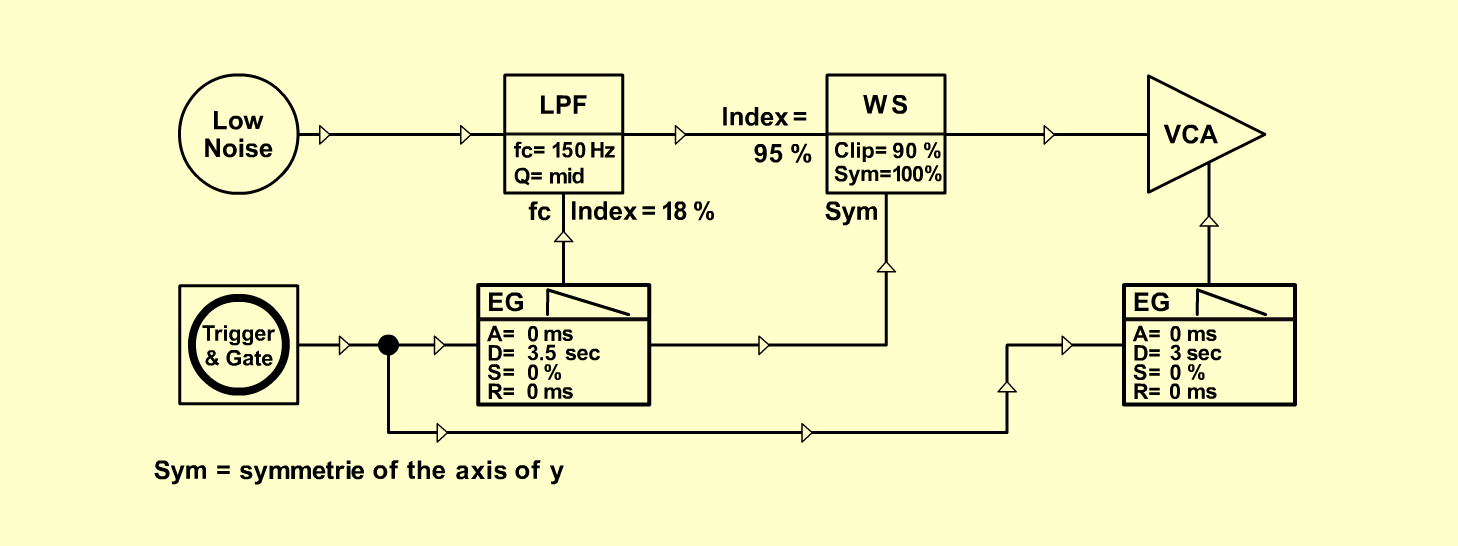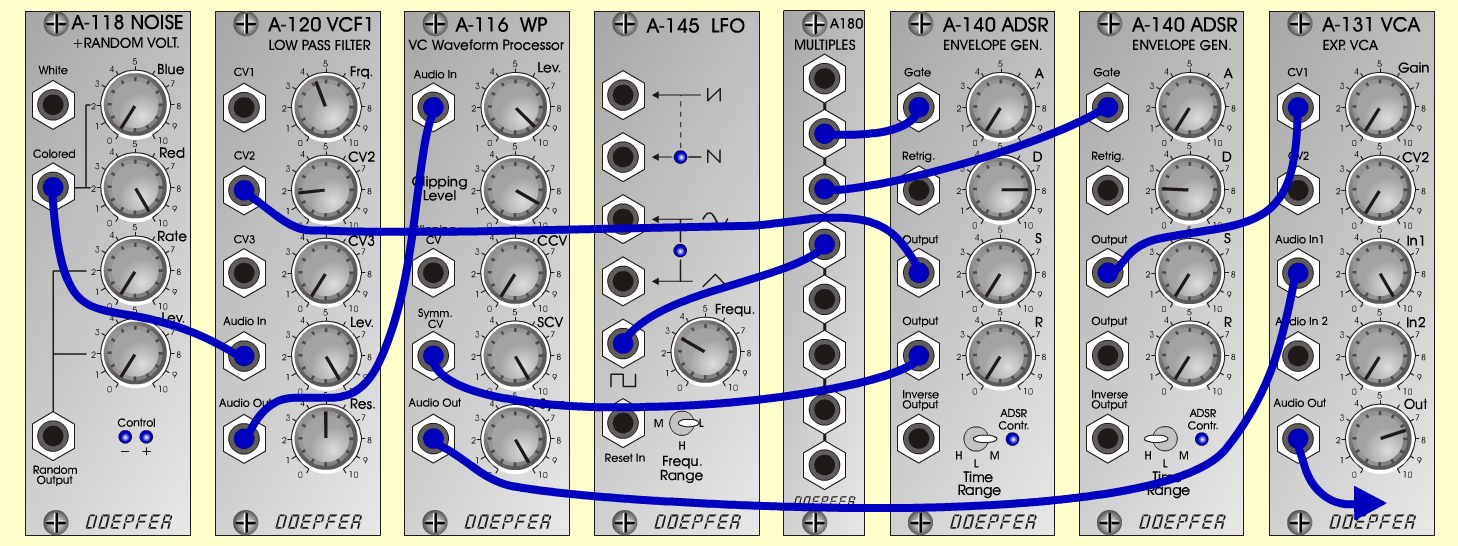ExplosionFXD o e p f e r A - 100 Connections: Settings: A-118 (Colored) <=>  A-120 (Audio In)   A-140/1 (Output) <=>  A-120 (CV 2)   A-140/1 (Output) <=> A-116 (Sym CV)   A-120 (Audio Out) <=> A-116 (Audio In)   A-116 (Audio Out) <=>  A-131 (Audio In1)   A-140/2 (Output) <=>  A-131 (CV 1)   Trigger and Gate: KB, Seq or   A-145 (Square) <=>  A-180   A-180 <=>  A-140/1 (Gate)   A-180 <=>  A-140/2 (Gate) A-118 (Blue = 0, Red = 10)   A-120 (Freq = 4.3, CV2 = 1.8, Lev = 10, Res = 5)   A-116 (Lev = 9.5, Clip = 9, CCV = 0, SCV = 10,   Sym = 10)   A-131 (Gain = 0, Audio In 1 = 10,   Audio Out = 7.4)   A-140/1 (A = 0, D = 8, S = 0, R = 0, Range = M)   A-140/2 (A = 0, D = 2.1, S = 0, R = 0, Range = H)   A-145 (Frq = 3, Range = L) Keld Sørensen, November 2000Sound sample Explosion